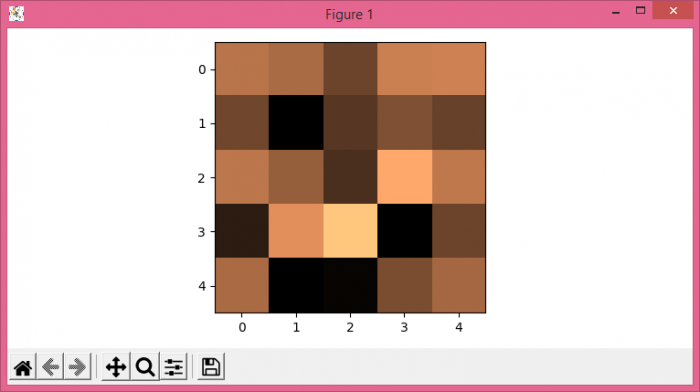# How can I get pyplot images to show on a console app? (Matplotlib)

To show pyplot images on a console, we can use pyplot.show() method.

## Steps

• Set the figure size and adjust the padding between and around the subplots.
• Create random data of 5☓5 dimension.
• Use imshow() method, with data. Display the data as an image, i.e., on a 2D regular raster.
• To display the figure, use show() method.

## Example

import numpy as np
from matplotlib import pyplot as plt

plt.rcParams["figure.figsize"] = [7.50, 3.50]
plt.rcParams["figure.autolayout"] = True

data = np.random.rand(5, 5)

plt.imshow(data, cmap="copper")

plt.show()

## Output